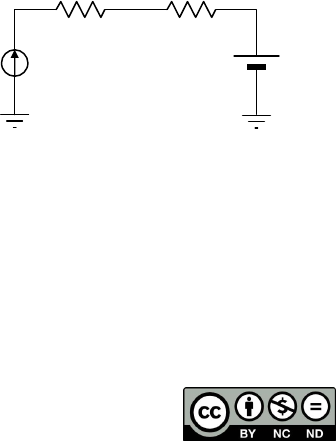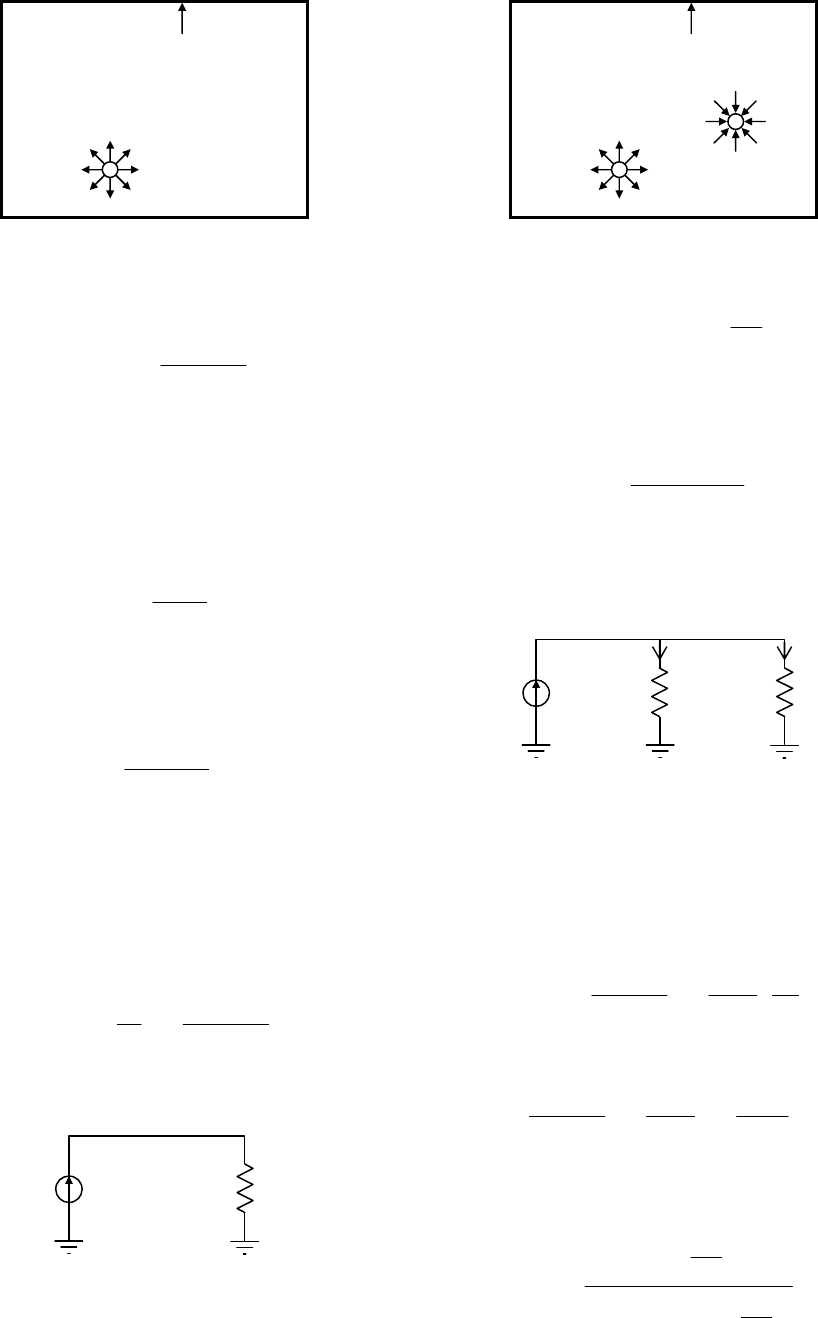Circuit Theory Analysis
of Acoustically Coupled Rooms
Análisis circuital de espacios acústicamente acoplados
Federico Miyara
Laboratorio de Acústica y Electroacústica, Facultad de Ciencias Exactas, Ingeniería y Agrimensura (FCEIA),
Riobamba 245 bis, Rosario, Argentina
fmiyara@fceia.unr.edu.ar
Abstract A circuit theory approach is presented for the
anal
ysis of acoustically coupled spaces. It is based on energy
flow and balance with a similar analogy to the one used for
solving dynamic thermal systems, where acoustic power is ana-
log to electric current and acoustic energy density is analog to
voltage. Room volume is equivalent to capacitor and sound
absorption is equivalent to a resistor. From these analogies and
Laplace-transformed models it is possible to get stationary or
transient response solutions.
Keywords: coupled rooms; circuit model; analogies
Resumen Se propone un enfoque circuital basado en
flujos de energía para el análisis de espacios acústicamente
acoplados. En el mismo se establece una analogía similar a la
que se utiliza en el estudio de sistemas térmicos dinámicos en el
cual la potencia acústica es análoga a la corriente eléctrica y la
densidad de energía acústica es análoga a la tensión. Los volú-
menes son equivalentes a capacitores y la absorción sonora es
asimilable a una resistencia. A partir de estas analogías se pue-
den plantear ecuaciones diferenciales y modelos en transfor-
mada de Laplace y obtener tanto soluciones estacionarias o de
régimen permanente como transitorias.
Palabras clave: recintos acoplados; modelo circuital; analogías
I. INTRODUCTION
Physi
cal systems can be represented by different formal
frameworks. Some of them are block diagrams, graph
theory  and bond graphs . Often the choice of one or
another depends on the nature of the process and on the kind
of the desired results. In the case of electric networks, cir-
cuit theory, based on Ohm’s and Kirchhoff’s laws, provides
an adequate approach because of the simplicity of the
graphical representation akin to the actual topology of the
physical system, and the availability of methods to write
down the equations by simple inspection. The use of the
Laplace transform allows to easily analyze circuits with
dynamic components such as capacitors and inductors.
The degree of maturity of circuit theory and the familiarity
with its techniques reached by electrical engineers and other
practitioners has prompted the extension of its principles to
other specialties such as hydraulics, mechanics and acoustics
. The general approach is to select two relevant variables
that can be considered analogous to voltage and electric
current and identify physical subprocesses where these
variables are linked. In general, but not always, the variables
are chosen so that their product has dimension of power. This
allows, by means of conservative transducers, the transition
from a physical domain to another, such as is the case of
electroacoustic equivalent circuits .
For instance, in the case of mechanical circuits, the
variables are force and velocity, and in the case of acoustic
or hydraulic systems, pressure and flow rate (or volume
velocity). In general there is a flow type variable and a
force type variable, which are the analogs to current and
voltage respectively.
Our purpose is to introduce an equivalent-circuit approach
applicable to the analysis of acoustically coupled rooms in
both static and dynamic conditions.
II. ANALOGIES
In th
e case of acoustically coupled spaces which we shall
be dealing with, we will take the acoustic power as the analog
of current. Should we stick to the rule that the product of the
two variables has dimension of power, the second variable
should be non-dimensional, which is not convenient.
We have the precedent of thermal circuits, in which the
relevant circuit variables are power and temperature ,
whose product has not dimension of power. They are often
used to estimate the working temperature of electronic
components (Fig. 1).
Fig. 1. An example of thermal circuit in which there is a power source (the
junct
ion), the thermal resistance between the junction and the case, R
T jc
,
the thermal resistance between the case and the environment, R
T jc
, and a
fixed ambient temperature, T
a
.
In order to select the force variable it will be useful to
analy
ze a simple case in steady state (Fig. 2).
In this example the reverberant field equation holds:
T
a
R
T jc
R
T ca
P
a
j
c
Revista elektron, Vol. 3, No. 1, pp. 1-5 (2019)
ISSN 2525-0159Fig. 2. A reverberant room in steady state with an acoustic power source W.
The power W
abs
absorbed at the surfaces and the square effective pressure
P
ef
2
of the reverberant field are shown. V is the volume, S, the surface, and
α, the absorption coefficient of the room.
W
S
cP
α
α
ρ=
)1(4
o
2
ef
(1)
where α is the mean sound absorption coefficient, W the
acoustic power, S the inner area of the room and ρ
o
c the
specific acoustic impedance of air .
While we could use P
ef
2
as the analog of voltage, it is
more convenient to use the acoustic energy density D,
which is proportional to P
ef
2
:
2
o
2
ef
c
P
D
ρ
= . (2)
This formula is valid for plane waves , but a diffuse field as
we are assuming is the superposition of a large number of
incoherent plane waves. Thus,
W
Sc
D
α
α
=
)1(4
. (3)
Given the analogy
D
V
W
I
we can define an analog to electric resistance, which we
shall call absorption resistance, as
Sc
W
D
R
α
α
==
)1(4
abs
. (4)
Now we can draw the first circuit for the reverberant steady
state (Fig.3).
Fig. 3. Circuit of the reverberant steady state in a room.
In the previous example the air absorption, which is
imp
ortant for large rooms, was not taken into account. In
this case (Fig. 4) α can be replaced by a coefficient α
tot
giv
en by
F
i
g
. 4. A reverberant room in steady state, considering the acoustic power
absorbed by the air.
S
V4
mtot
γ+α=α
. (5)
where V is the volume, S the area and γ
m
, the coefficient of
absorption in the air, in neper/m . Then
Sc
R
tot
tot
)1(4
α
α
= . (6)
It is interesting to note that this resistance can be considered
as the parallel of two resistances, one of them representing
the absorption by the surfaces, and the other, by the air.
Fig. 5. Equivalent circuit for the steady state of the reverberant field in a
room, including the absorption in the air.
Bec
ause of energy conservation, we have an equivalent of
Kirchhoff’s first law,
absair
WWW
= . (7)
D
Sc
W
411
tot
tot
air
α
α
α
α
=
. (8)
We can look for a value α' such that
'1
'
11
tot
tot
α
α
=
α
α
α
α
. (9)
This value represents a hypothetical surface absorption equiva-
lent to air absorption. Solving for α'
αγ+α
γ
=α
S
V
S
V
4
)1(
4
'
m
2
m
. (10)
The
distinction between both absorption mechanisms is of
conceptual interest and also illustrates a direct application of
P
ef
2
V, S,
α
W
W
abs
R
abs
W
D
+
P
ef
2
V
1
, S
1
,
α
1
W
W
air
W
abs
R
abs
W
D
+
R
air
W
air
W
abs
Revista elektron, Vol. 3, No. 1, pp. 1-5 (2019)
ISSN 2525-0159
http://elektron.fi.uba.ar